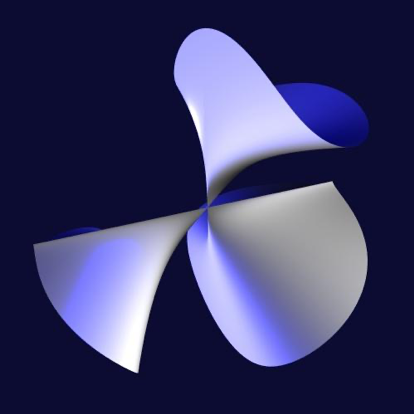003

# STAG

## Singularity Theory and Algebraic Geometry

Develop new methods in Singularity Theory designed in order to approach the solution of several classical conjectures that have resisted current methods in singularity theory, and explore new interactions of singularities with the latest developments in nearby areas.

Singularities arise naturally in a huge number of different areas of mathematics and science. As a consequence Singularity Theory lies at the crossroads of the paths connecting applications of mathematics with its most abstract parts. For example, it connects the investigation of optical caustics with simple Lie algebras and regular polyhedra theory, while also relating hyperbolic PDE wavefronts to knot theory and the theory of the shape of solids to commutative algebra.

The main goal in most problems of singularity theory is to understand the dependence of some objects of analysis and geometry, or physics, or from some other science on parameters. For generic points in the parameter space their exact values influence only the quantitative aspects of the phenomena, their qualitative, topological features remaining stable under small changes of parameter values.

However, for certain exceptional values of the parameters these qualitative features may suddenly change under a small variation of the parameter. This change is called a perestroika, bifurcation or catastrophe in different branches of the sciences. A typical example is that of Morse surgery, describing the perestroika of the level variety of a function as the function crosses through a critical value. (This has an important complex counterpart - the Picard-Lefschetz theory concerning the branching of integrals.) Other familiar examples include caustics and outlines or profiles of surfaces obtained from viewing or projecting from a point, or in a given direction.

Algebraic geometry classically studies solutions of systems of polynomial equations in several variables multivariate polynomials. It is even more important to understand the intrinsic and geometric properties of the totality of solutions of a system of equations, than to find a specific solution.

It is is based on the use and the combinations of abstract algebraic techniques, mainly from commutative algebra, and analytic techniques, mainly from complex variables theory and complex differential geometry. Recently deep interactions with gauge theory are also at the core of current research.

The fundamental objects of study in algebraic geometry are algebraic varieties. Basic questions involve the study of geometry and topology, both globally and locally near the points of special interest like the singular points, the inflection points...

Algebraic geometry has deep connections with other parts of pure mathematics, like topology, number theory or differential geometry. Despite being a part of pure mathematics it has found important applications in criptography, coding theory and physics (it is at the core of string theory, for example).

Our research: We focus mainly in Singularities appearing in Algebraic Geometry developing the theoretical core of Singularity Theory and its interactions and applications to Complex and Algebraic Geometry, Differential/symplectic/Contact Topology, and Hodge Theory.Milnor fibration for the cuspidal surve singularity.

The author of the video is Miguel Marco Buzunariz

Siingularity theory has exchanged methods with Hodge theory, D-modules, and perverse sheaves, algebraic and geometric topology or algebra. Important classical questions remain unresolved and resist current methods. New trends and techniques in singularity theory have been incorporated recently. Part of them arose from new major developments in geometry in the last years, that are bringing new methods, ideas and questions, and ultimately reshaping singularity theory: non-arquimedean methods, symplectic topology, gauge theories,  the Minimal Model Program, mirror symmetry and new developments around the decomposition theorem are important examples.  But also the study of singularities from the Lipschitz geometry view point (a development that is taking place mainly within singularity theory) is one of the most flourishing trends, which probably will transcend the borders of the singularity theory and influence other areas in the future.

The topics described above indicate our sources of inspiration when we approach research, but obviously we do not pursue  actively such a  width of topics.  At the present moment, together with several sets of cooperators, we are carrying active projects and thinking in the following lines (some of them are reflected by our preprints in the arXiv):

1. Study of the connection between Floer homology of iterates of the monodromy of a hypersurface with the ordinary cohomology of contact loci. The study of contact loci connects with the study of monodromy conjecture, where active thinking is also being carried
2. Study of simultaneous resolution deformations, potentially in connection with the variation of the Heegard-Floer homology of the link.Study of Heegard-Floer homology of links via Nemethi's Lattice Cohomology
3. Developing invariants able to capture Lipschitz geometry phenomena of singularities. Concretely we are pursuing the development of Moderately Discontinuous Homology.
4. Applications of the Decomposition Theorem to characteristic classes of singular varieties, and to several classical questions.
5. Study of Maximal Cohen Macaulay modules, singularities categories and matrix factorizations.
6. Study of zeta functions associated with singularities, towards a better understanding of the Monodromy Conjecture.x3+y2z3+yz4=0 x2-y2z2=0

Figures above are some Algebraic Surface examples

The author of the images is Herwing Hauser

STAG
Name: Pallares, Irma
University: University of the Basque Country (UPV/EHU)
STAG

## Moderately Discontinuous Algebraic Topology for Metric Subanalytic Germs

Name: Heinze, Sonja
University: Autonomus University of Madrid (UAM)
STAG

## Monodromies as Têtê-à-Têtê graphs

Name: Portilla, Pablo